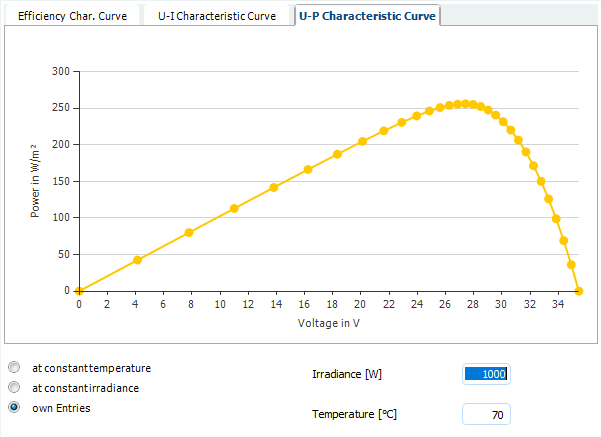# calculation of Min. MPP Voltage

## Recommended Posts

Hello,
Could you say what is the methodology for calculating the minimum voltage for MPP??
I add print screen for calcuation made by PVSol for Fronius ECO 27 and LONGi HPH 320 (both products from build-in database)Why the min MPP is so low?

My reasoning:
Tvoc = -0,286
deltaT = 70-25 = 45
45*(-0,286) = ~ -13%

Vmpp = 33,9
Vmpp at 70°C = 33,9 - (33,9* 0,13) = 33,9 - 4,41 = 29,49
20*29,49 = 589,8

Calculation from Fronius Solar Configurator:##### Share on other sites

Hello Infinitech,

very good question, and an important one!

It is unfortunately a common mistake, even among experts in the community, to mix up the temperature coefficients of the open circuit and MPP voltage. These are not the same!

The LR6-60 HPH 320M module in our database has a temperature coefficient for the open circuit voltage of -116.97 mV/K, or 0.286 %/K. So the Voc at 70°C will be

Voc(70°C) = Voc(25°C) + 45 K * (-0.117 V/K) = 40,9 V - 5.265 V = 35.64 V

The temperature coefficient of the MPP voltage will be different, however, usually higher than for Voc. You can easily see that the temperature coefficient of the MPP voltage must be higher than the one of Voc by looking at the temperature coefficient of the power, which is -0.37 %/K. So there must be additional, temperature related losses to close the gap between -0.37 % and -0.286 %.

This is why we have to simulate the module at the given temperatures we want to analyse. We take the three temperature coefficients of Voc, Isc and Pmpp and simulate the module characteristics at a given temperature, e.g. 70°C. From these curves we can detect MPP voltage and current.

See here the diagram of the power over voltage for different temperatures for the LR6-60 HPH 320M module. Voc(70°C) is around 35V, as expected. The MPP voltage seems to be at 27.5 V, which relates to a relative loss of -0.425 %/K. This is where the 551 V come from, 20 * 27.5 V ≈ 551 V.The other way 'round, you can easily check that an Vmpp of 590.74 V (as stated by Fronius) must be too high to meet the condition of the temperature coefficient of the power.

Simple check: Vmpp(70°C) = 590.74 V, Impp(70°C) = 9.69 A, so the Pmpp(70°C) would be 590.74 V * 9.69 A = 5722 W. This would be a relative change of -0.233 %/K of the power, so far too less. The datasheet says -0.37 %/K.

Hope that clarifies the matter. If you have any question on the topic, please don't hesitate to ask. In the meanwhile, we will contact Fronius to point them out to this problem as well.

Kind regards,

Martin

##### Share on other sites

Dear developer_mh,

Can you please advise how then can we get the Vmpp temp. coefficient, and if only the Voc temp. coefficient is available in the data sheet can we determine the Vmpp temp. coefficient out of it?

As per the NEC NFPA70 A.690 and some practices, it's accepted to consider the Pmax. temp. coefficient in case Vmpp temp. coef. is not available. Is this correct?

While as per RENAC installation training if the Vmpp temp. coeff. is not available then consider the Voc one (either in mV or %/C). Kindly advise?

##### Share on other sites

Hello Ragy,

I would recommend to use the software (e.g. PV*SOL) to simulate the MPP voltage for a given temperature. In my opinion it is not possible to calculate it with easy formulas.

If only the temperature coefficient (tc) of Voc and Pmpp are given on the data sheet, there is no way (known to me) to derive from those numbers the tc of Vmpp, except by simulating.

Quote

it's accepted to consider the Pmax. temp. coefficient in case Vmpp temp. coef. is not available

Quote

if the Vmpp temp. coeff. is not available then consider the Voc one

I'd guess that the tc of Pmpp (Pmax) (in %) is always closer to the tc of Vmpp than to the tc of Voc. So, if you can't simulate, choose the tc of Pmpp.

But really, in the end it is those questions why we need simulation tools in order to plan our PV systems correctly. If you want to calculate in your head, then this is ok, but be aware that the results will not be reliable. We do not recommend any other method to determine the voltage ranges of PV systems other than simulating.

Kind regards,

Martin

##### Share on other sites

Dear Martin,

Thank you so much for your useful reply. Today, you learnt me something new!

I use licensed PVSol since months and I'm a big fan of it, but I thought a formula for this particular calculation may be easily derived. I decided already that final PV systems planning shall be done only by the software. However, in case modules manufacturers know the Vmpp temp. Coefficient is it then accurate to use it or still simulation give better results?

Thank you again

##### Share on other sites

Hi Ragy,

I guess that if module manufacturers know the tc of Vmpp and publish it, you are safe to use it for calculation.

Kind regards,

Martin

## Join the conversation

You can post now and register later. If you have an account, sign in now to post with your account.×   Pasted as rich text.   Paste as plain text instead

Only 75 emoji are allowed.

×   Your previous content has been restored.   Clear editor

×   You cannot paste images directly. Upload or insert images from URL.

×
×